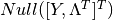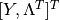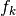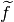# crnt4sbml.MassConservationApproach¶

class `crnt4sbml.``MassConservationApproach`(cgraph, get_physiological_range)[source]

Class for constructing variables and methods needed for the mass conservation approach.

`__init__`(cgraph, get_physiological_range)[source]

Initialization of the MassConservationApproach class.

Methods

 `__init__`(cgraph, get_physiological_range) Initialization of the MassConservationApproach class. `generate_report`() Prints out helpful details constructed by `crnt4sbml.MassConservationApproach.run_optimization()` and `crnt4sbml.MassConservationApproach.run_continuity_analysis()`. `get_conservation_laws`() Returns a string representation of the conservation laws. `get_decision_vector`() Returns a list of SymPy variables that represent the decision vector of the optimization problem. `get_objective_fun_params`() Returns a list of SymPy variables that represent those variables that may be contained in the G matrix, Jacobian of the equilibrium manifold with respect to the species, or objective function. `get_concentration_vals`() Returns a list of SymPy expressions representing the species in terms of those variables present in the decision vector. `get_concentration_solutions`() Returns a more readable string representation of the species defined in terms of the decision vector. `get_concentration_funs`() Returns a list of lambda functions representing each of the species. `get_concentration_bounds_species`() Returns a list of SymPy variables that represents the order of species for the concentration bounds provided to `crnt4sbml.MassConservationApproach.run_optimization()`. `get_w_nullspace`() Returns a list of SymPy column vectors representing. `get_w_matrix`() Returns SymPy matrix, which we call the W matrix. `get_dch_matrix`() Returns a SymPy matrix representing the Jacobian of the equilibrium manifold with respect to the species. `get_lambda_dch_matrix`() Returns a lambda function representation of the Jacobian of the equilibrium manifold matrix. `get_h_vector`() Returns a SymPy matrix representing the equilibrium manifold. `get_g_matrix`() Returns a SymPy matrix representing the G matrix of the defined optimization problem. `get_lambda_g_matrix`() Returns a lambda function representation of the G matrix. `get_symbolic_objective_fun`() Returns SymPy expression for the objective function of the optimization problem. `get_lambda_objective_fun`() Returns a lambda function representation of the objective function of the optimization problem. `get_independent_odes`() Returns a SymPy Matrix where the rows represent the independent ODEs used in the numerical continuation routine. `get_independent_species`() Returns a list of SymPy representations of the independent species used in the numerical continuation routine. `get_optimization_bounds`() Builds all of the necessary physiological bounds for the optimization routine. `get_my_rank`() Returns the rank assigned by mpi4py if it is initialized, otherwise None will be returned. `get_comm`() Returns a mpi4py communicator if it has been initialized and None otherwise. `run_optimization`([bounds, iterations, …]) Function for running the optimization problem for the mass conservation approach. `run_continuity_analysis`([species, …]) Function for running the numerical continuation and bistability analysis portions of the mass conservation approach. `run_greedy_continuity_analysis`([species, …]) Function for running the greedy numerical continuation and bistability analysis portions of the mass conservation approach. `run_direct_simulation`([response, signal, …]) Function for running direct simulation to conduct bistability analysis of the mass conservation approach.
`generate_report`()[source]

Prints out helpful details constructed by `crnt4sbml.MassConservationApproach.run_optimization()` and `crnt4sbml.MassConservationApproach.run_continuity_analysis()`.

Example

`get_comm`()[source]

Returns a mpi4py communicator if it has been initialized and None otherwise.

`get_concentration_bounds_species`()[source]

Returns a list of SymPy variables that represents the order of species for the concentration bounds provided to `crnt4sbml.MassConservationApproach.run_optimization()`. `Fig1Ci.xml` for the provided example.

Example

```>>> import crnt4sbml
>>> network = crnt4sbml.CRNT("path/to/Fig1Ci.xml")
>>> approach = network.get_mass_conservation_approach()
Creating Equilibrium Manifold ...
Elapsed time for creating Equilibrium Manifold: 2.060944
```
```>>> print(approach.get_concentration_bounds_species())
[s1, s3, s7, s16]
```
`get_concentration_funs`()[source]

Returns a list of lambda functions representing each of the species. Here the species are those expressions provided by `crnt4sbml.MassConservationApproach.get_concentration_vals()` where the arguments of each lambda function is provided by `crnt4sbml.MassConservationApproach.get_decision_vector()`. `Fig1Ci.xml` for the provided example.

Example

```>>> import crnt4sbml
>>> network = crnt4sbml.CRNT("path/to/Fig1Ci.xml")
>>> approach = network.get_mass_conservation_approach()
Creating Equilibrium Manifold ...
Elapsed time for creating Equilibrium Manifold: 2.060944
```
```>>> print(approach.get_concentration_funs())
[<function _lambdifygenerated at 0x135f8b4d0>, <function _lambdifygenerated at 0x135f72050>,
<function _lambdifygenerated at 0x135f728c0>, <function _lambdifygenerated at 0x135f725f0>,
<function _lambdifygenerated at 0x135f5f830>, <function _lambdifygenerated at 0x135fa0170>,
<function _lambdifygenerated at 0x135fa04d0>]
```
`get_concentration_solutions`()[source]

Returns a more readable string representation of the species defined in terms of the decision vector. `Fig1Ci.xml` for the provided example.

Example

```>>> import crnt4sbml
>>> network = crnt4sbml.CRNT("path/to/Fig1Ci.xml")
>>> approach = network.get_mass_conservation_approach()
Creating Equilibrium Manifold ...
Elapsed time for creating Equilibrium Manifold: 2.060944
```
```>>> print(approach.get_concentration_solutions())
s1 = s15*(re5r + re6)/(re5*s6)
s2 = s2
s3 = re1*s15*s2*(re5r + re6)/(re5*s6*(re1r + re2))
s6 = s6
s7 = -s15*(re5*re5r*s6*(re1r + re2)*(re3r + re4) - (re5r + re6)*(-re1*re1r*re3r*s2 - re1*re1r*re4*s2 + re1*re3r*s2*(re1r + re2) + re1*re4*s2*(re1r + re2) + re5*s6*(re1r + re2)*(re3r + re4)))/(re3*re4*re5*s6**2*(re1r + re2))
s16 = s15*(re1*re2*re5r*s2 + re1*re2*re6*s2 + re1r*re5*re6*s6 + re2*re5*re6*s6)/(re4*re5*s6*(re1r + re2))
s15 = s15
```
`get_concentration_vals`()[source]

Returns a list of SymPy expressions representing the species in terms of those variables present in the decision vector. The order is that established in `crnt4sbml.Cgraph.get_species()`. Note that if only a single species is provided as an element in the list, this means the species is a free variable.

`Fig1Ci.xml` for the provided example.

Example

```>>> import crnt4sbml
>>> network = crnt4sbml.CRNT("path/to/Fig1Ci.xml")
>>> approach = network.get_mass_conservation_approach()
Creating Equilibrium Manifold ...
Elapsed time for creating Equilibrium Manifold: 2.060944
```
```>>> print(approach.get_concentration_vals())
[s15*(re5r + re6)/(re5*s6), s2, re1*s15*s2*(re5r + re6)/(re5*s6*(re1r + re2)), s6,
-s15*(re5*re5r*s6*(re1r + re2)*(re3r + re4) - (re5r + re6)*(-re1*re1r*re3r*s2 - re1*re1r*re4*s2 +
re1*re3r*s2*(re1r + re2) + re1*re4*s2*(re1r + re2) + re5*s6*(re1r + re2)*(re3r + re4)))/(re3*re4*re5*s6**2*
(re1r + re2)), s15*(re1*re2*re5r*s2 + re1*re2*re6*s2 + re1r*re5*re6*s6 + re2*re5*re6*s6)/(re4*re5*s6*(re1r + re2)), s15]
```
`get_conservation_laws`()[source]

Returns a string representation of the conservation laws. Here the values on the left hand side of each equation are the constants of the conservation laws. `Fig1Ci.xml` for the provided example.

Example

```>>> import crnt4sbml
>>> network = crnt4sbml.CRNT("path/to/Fig1Ci.xml")
>>> approach = network.get_mass_conservation_approach()
Creating Equilibrium Manifold ...
Elapsed time for creating Equilibrium Manifold: 2.060944
```
```>>> print(approach.get_conservation_laws())
C1 = 1.0*s16 + 1.0*s7
C2 = 1.0*s2 + 1.0*s3
C3 = 1.0*s1 + 2.0*s15 + 1.0*s16 + 1.0*s3 + 1.0*s6
```
`get_dch_matrix`()[source]

Returns a SymPy matrix representing the Jacobian of the equilibrium manifold with respect to the species. `Fig1Ci.xml` for the provided example.

Example

```>>> import crnt4sbml
>>> import sympy
>>> network = crnt4sbml.CRNT("path/to/Fig1Ci.xml")
>>> approach = network.get_mass_conservation_approach()
Creating Equilibrium Manifold ...
Elapsed time for creating Equilibrium Manifold: 2.060944
```
```>>> sympy.pprint(approach.get_dch_matrix())
⎡-re₁⋅s₂  -re₁⋅s₁     re1r         0        0          0            0     ⎤
⎢                                                                         ⎥
⎢re₁⋅s₂   re₁⋅s₁   -re1r - re₂     0        0          0            0     ⎥
⎢                                                                         ⎥
⎢   0        0          0       -re₃⋅s₇  -re₃⋅s₆     re3r           0     ⎥
⎢                                                                         ⎥
⎢   0        0          0       re₃⋅s₇   re₃⋅s₆   -re3r - re₄       0     ⎥
⎢                                                                         ⎥
⎢-re₅⋅s₆     0          0       -re₅⋅s₁     0          0          re5r    ⎥
⎢                                                                         ⎥
⎣re₅⋅s₆      0          0       re₅⋅s₁      0          0       -re5r - re₆⎦
```
`get_decision_vector`()[source]

Returns a list of SymPy variables that represent the decision vector of the optimization problem. `Fig1Ci.xml` for the provided example.

Example

```>>> import crnt4sbml
>>> network = crnt4sbml.CRNT("path/to/Fig1Ci.xml")
>>> approach = network.get_mass_conservation_approach()
Creating Equilibrium Manifold ...
Elapsed time for creating Equilibrium Manifold: 2.060944
```
```>>> print(approach.get_decision_vector())
[re1, re1r, re2, re3, re3r, re4, re5, re5r, re6, s2, s6, s15]
```
`get_g_matrix`()[source]

Returns a SymPy matrix representing the G matrix of the defined optimization problem. `Fig1Ci.xml` for the provided example.

Example

```>>> import crnt4sbml
>>> import sympy
>>> network = crnt4sbml.CRNT("path/to/Fig1Ci.xml")
>>> approach = network.get_mass_conservation_approach()
Creating Equilibrium Manifold ...
Elapsed time for creating Equilibrium Manifold: 2.060944
```
```>>> sympy.pprint(approach.get_g_matrix())
⎡-re₁⋅s₂  -re₁⋅s₁     re1r         0        0          0            0       1  -1⎤
⎢                                                                                ⎥
⎢re₁⋅s₂   re₁⋅s₁   -re1r - re₂     0        0          0            0       0  0 ⎥
⎢                                                                                ⎥
⎢   0        0          0       -re₃⋅s₇  -re₃⋅s₆     re3r           0       1  0 ⎥
⎢                                                                                ⎥
⎢   0        0          0       re₃⋅s₇   re₃⋅s₆   -re3r - re₄       0       0  0 ⎥
⎢                                                                                ⎥
⎢-re₅⋅s₆     0          0       -re₅⋅s₁     0          0          re5r      0  1 ⎥
⎢                                                                                ⎥
⎢re₅⋅s₆      0          0       re₅⋅s₁      0          0       -re5r - re₆  0  0 ⎥
⎢                                                                                ⎥
⎢   0        0          0          0       1.0        1.0           0       0  0 ⎥
⎢                                                                                ⎥
⎢   0       1.0        1.0         0        0          0            0       0  0 ⎥
⎢                                                                                ⎥
⎣  1.0       0         1.0        1.0       0         1.0          2.0      0  0 ⎦
```
`get_h_vector`()[source]

Returns a SymPy matrix representing the equilibrium manifold. `Fig1Ci.xml` for the provided example.

Example

```>>> import crnt4sbml
>>> import sympy
>>> network = crnt4sbml.CRNT("path/to/Fig1Ci.xml")
>>> approach = network.get_mass_conservation_approach()
Creating Equilibrium Manifold ...
Elapsed time for creating Equilibrium Manifold: 2.060944
```
```>>> sympy.pprint(approach.get_h_vector())
⎡a₁ - a₂ - re₁⋅s₁⋅s₂ + re1r⋅s₃⎤
⎢                             ⎥
⎢re₁⋅s₁⋅s₂ + s₃⋅(-re1r - re₂) ⎥
⎢                             ⎥
⎢  a₁ - re₃⋅s₆⋅s₇ + re3r⋅s₁₆  ⎥
⎢                             ⎥
⎢re₃⋅s₆⋅s₇ + s₁₆⋅(-re3r - re₄)⎥
⎢                             ⎥
⎢  a₂ - re₅⋅s₁⋅s₆ + re5r⋅s₁₅  ⎥
⎢                             ⎥
⎣re₅⋅s₁⋅s₆ + s₁₅⋅(-re5r - re₆)⎦
```
`get_independent_odes`()[source]

Returns a SymPy Matrix where the rows represent the independent ODEs used in the numerical continuation routine. Here the entries of the list correspond to the time derivatives of the corresponding species provided by `crnt4sbml.MassConservationApproach.get_independent_species()`. Note that the independent ODEs created are based on the species chosen for the numerical continuation. Thus, the continuation routine needs to be ran first. If this function is called before the numerical continuation routine then None will be returned.

Example

```>>> import crnt4sbml
>>> network = crnt4sbml.CRNT("path/to/sbml_file.xml")
>>> approach = network.get_mass_conservation_approach()
>>> multistable_param_ind = approach.run_greedy_continuity_analysis(species="species", parameters=params_for_global_min,
auto_parameters={'PrincipalContinuationParameter': "PCP"})
>>> odes = approach.get_independent_odes()
```
`get_independent_species`()[source]

Returns a list of SymPy representations of the independent species used in the numerical continuation routine. Note that the independent species created are based on the species chosen for the numerical continuation. Thus, the continuation routine needs to be ran first. If this function is called before the numerical continuation routine then None will be returned.

Example

```>>> import crnt4sbml
>>> network = crnt4sbml.CRNT("path/to/sbml_file.xml")
>>> approach = network.get_mass_conservation_approach()
>>> multistable_param_ind = approach.run_greedy_continuity_analysis(species="species", parameters=params_for_global_min,
auto_parameters={'PrincipalContinuationParameter': "PCP"})
>>> species = approach.get_independent_species()
```
`get_lambda_dch_matrix`()[source]

Returns a lambda function representation of the Jacobian of the equilibrium manifold matrix. Here the arguments of the lambda function are given by the values provided by `crnt4sbml.MassConservationApproach.get_objective_fun_params()`. `Fig1Ci.xml` for the provided example.

Example

```>>> import crnt4sbml
>>> network = crnt4sbml.CRNT("path/to/Fig1Ci.xml")
>>> approach = network.get_mass_conservation_approach()
Creating Equilibrium Manifold ...
Elapsed time for creating Equilibrium Manifold: 2.060944
```
```>>> print(approach.get_lambda_dch_matrix())
<function _lambdifygenerated at 0x131a06ea0>
```
`get_lambda_g_matrix`()[source]

Returns a lambda function representation of the G matrix. Here the arguments of the lambda function are given by the values provided by `crnt4sbml.MassConservationApproach.get_objective_fun_params()`. `Fig1Ci.xml` for the provided example.

Example

```>>> import crnt4sbml
>>> network = crnt4sbml.CRNT("path/to/Fig1Ci.xml")
>>> approach = network.get_mass_conservation_approach()
Creating Equilibrium Manifold ...
Elapsed time for creating Equilibrium Manifold: 2.060944
```
```>>> print(approach.get_lambda_g_matrix())
<function _lambdifygenerated at 0x13248ac80>
```
`get_lambda_objective_fun`()[source]

Returns a lambda function representation of the objective function of the optimization problem. Here the arguments of the lambda function are given by the values provided by `crnt4sbml.MassConservationApproach.get_objective_fun_params()`. `Fig1Ci.xml` for the provided example.

Example

```>>> import crnt4sbml
>>> network = crnt4sbml.CRNT("path/to/Fig1Ci.xml")
>>> approach = network.get_mass_conservation_approach()
Creating Equilibrium Manifold ...
Elapsed time for creating Equilibrium Manifold: 2.060944
```
```>>> print(approach.get_lambda_objective_fun())
<function _lambdifygenerated at 0x12f6f7ea0>
```
`get_my_rank`()[source]

Returns the rank assigned by mpi4py if it is initialized, otherwise None will be returned.

`get_objective_fun_params`()[source]

Returns a list of SymPy variables that represent those variables that may be contained in the G matrix, Jacobian of the equilibrium manifold with respect to the species, or objective function. `Fig1Ci.xml` for the provided example.

Example

```>>> import crnt4sbml
>>> network = crnt4sbml.CRNT("path/to/Fig1Ci.xml")
>>> approach = network.get_mass_conservation_approach()
Creating Equilibrium Manifold ...
Elapsed time for creating Equilibrium Manifold: 2.060944
```
```>>> print(approach.get_objective_fun_params())
[re1, re1r, re2, re3, re3r, re4, re5, re5r, re6, s1, s2, s3, s6, s7, s16, s15]
```
`get_optimization_bounds`()[source]

Builds all of the necessary physiological bounds for the optimization routine. `Fig1Ci.xml` for the provided example.

Returns: bounds (list of tuples) – List of tuples defining the upper and lower bounds for the decision vector variables based on physiological ranges. concentration_bounds (list of tuples) – List of tuples defining the upper and lower bounds for those concentrations not in the decision vector based on physiological ranges.

Examples

```>>> import crnt4sbml
>>> network = crnt4sbml.CRNT("path/to/Fig1Ci.xml")
>>> approach = network.get_mass_conservation_approach()
Creating Equilibrium Manifold ...
Elapsed time for creating Equilibrium Manifold: 2.060944
```
```>>> bounds, concentration_bounds = approach.get_optimization_bounds()
>>> print(bounds)
[(1e-08, 0.0001), (1e-05, 0.001), (0.001, 1.0), (1e-08, 0.0001), (1e-05, 0.001), (0.001, 1.0),
(1e-08, 0.0001), (1e-05, 0.001), (0.001, 1.0), (0.5, 500000.0), (0.5, 500000.0), (0.5, 500000.0)]
```
```>>> print(concentration_bounds)
[(0.5, 500000.0), (0.5, 500000.0), (0.5, 500000.0), (0.5, 500000.0)]
```
`get_symbolic_objective_fun`()[source]

Returns SymPy expression for the objective function of the optimization problem. This is the determinant of the G matrix squared. `Fig1Ci.xml` for the provided example.

Example

```>>> import crnt4sbml
>>> network = crnt4sbml.CRNT("path/to/Fig1Ci.xml")
>>> approach = network.get_mass_conservation_approach()
Creating Equilibrium Manifold ...
Elapsed time for creating Equilibrium Manifold: 2.060944
```
```>>> print(approach.get_symbolic_objective_fun())
1.0*re1**2*re2**2*re3**2*re4**2*re5**2*re6**2*s1**2*s6**2*s7**2*((1.0*s2/s7 - 1.0*s2*(-re3r - re4)/
(re3*s6*s7))/re4 + (1.0 + 1.0*re1r/(re1*s1))/re2 + 1.0/(re1*s1))**2*(-((1.0*s6*(-1.0*s1/s6 + 1.0)/s7 +
1.0 - (-re3r - re4)*(-1.0*s1/s6 + 1.0)/(re3*s7))/re4 + 1.0/re2)*(-1.0*re5r*s2/(re5*re6*s1*s6) -
1.0*s2*(1 + re5*s6/(re1*s2))/(re5*s1*s6) - (1.0 + 1.0*re1r/(re1*s1))/re2)/((1.0*s2/s7 - 1.0*s2*
(-re3r - re4)/(re3*s6*s7))/re4 + (1.0 + 1.0*re1r/(re1*s1))/re2 + 1.0/(re1*s1)) + (2.0 + 1.0*re5r/(re5*s6))/
re6 + 1.0*(1 + re5*s6/(re1*s2))/(re5*s6) - 1.0/re2 - 1.0/(re1*s2))**2
```
`get_w_matrix`()[source]

Returns SymPy matrix, which we call the W matrix. `Fig1Ci.xml` for the provided example.

Example

```>>> import crnt4sbml
>>> import sympy
>>> network = crnt4sbml.CRNT("path/to/Fig1Ci.xml")
>>> approach = network.get_mass_conservation_approach()
Creating Equilibrium Manifold ...
Elapsed time for creating Equilibrium Manifold: 2.060944
```
```>>> sympy.pprint(approach.get_w_matrix())
⎡1  0  0  0  0  1  1  0  0⎤
⎢                         ⎥
⎢1  0  1  0  0  0  0  0  0⎥
⎢                         ⎥
⎢0  1  0  0  0  0  0  0  0⎥
⎢                         ⎥
⎢0  0  1  1  0  0  1  0  2⎥
⎢                         ⎥
⎢0  0  0  1  0  1  0  0  0⎥
⎢                         ⎥
⎢0  0  0  0  1  0  0  0  0⎥
⎢                         ⎥
⎢0  0  0  0  0  0  0  1  0⎥
⎢                         ⎥
⎢1  1  1  0  0  0  0  0  0⎥
⎢                         ⎥
⎢0  0  0  1  1  1  0  0  0⎥
⎢                         ⎥
⎣0  0  0  0  0  0  1  1  1⎦
```
`get_w_nullspace`()[source]

Returns a list of SymPy column vectors representing. `Fig1Ci.xml` for the provided example.

Example

```>>> import crnt4sbml
>>> import sympy
>>> network = crnt4sbml.CRNT("path/to/Fig1Ci.xml")
>>> approach = network.get_mass_conservation_approach()
Creating Equilibrium Manifold ...
Elapsed time for creating Equilibrium Manifold: 2.060944
```
```>>> sympy.pprint(approach.get_w_nullspace())
⎡⎡-1⎤  ⎡1 ⎤⎤
⎢⎢  ⎥  ⎢  ⎥⎥
⎢⎢0 ⎥  ⎢0 ⎥⎥
⎢⎢  ⎥  ⎢  ⎥⎥
⎢⎢1 ⎥  ⎢-1⎥⎥
⎢⎢  ⎥  ⎢  ⎥⎥
⎢⎢-1⎥  ⎢0 ⎥⎥
⎢⎢  ⎥  ⎢  ⎥⎥
⎢⎢0 ⎥, ⎢0 ⎥⎥
⎢⎢  ⎥  ⎢  ⎥⎥
⎢⎢1 ⎥  ⎢0 ⎥⎥
⎢⎢  ⎥  ⎢  ⎥⎥
⎢⎢0 ⎥  ⎢-1⎥⎥
⎢⎢  ⎥  ⎢  ⎥⎥
⎢⎢0 ⎥  ⎢0 ⎥⎥
⎢⎢  ⎥  ⎢  ⎥⎥
⎣⎣0 ⎦  ⎣1 ⎦⎦
```
`run_continuity_analysis`(species=None, parameters=None, dir_path='./num_cont_graphs', print_lbls_flag=False, auto_parameters=None, plot_labels=None)[source]

Function for running the numerical continuation and bistability analysis portions of the mass conservation approach.

Parameters: species (string) – A string stating the species that is the y-axis of the bifurcation diagram. parameters (list of numpy arrays) – A list of numpy arrays corresponding to the decision vectors that produce a small objective function value. dir_path (string) – A string stating the path where the bifurcation diagrams should be saved. print_lbls_flag (bool) – If True the routine will print the special points found by AUTO 2000 and False will not print any special points. auto_parameters (dict) – Dictionary defining the parameters for the AUTO 2000 run. Please note that one should not set ‘SBML’ or ‘ScanDirection’ in these parameters as these are automatically assigned. It is absolutely necessary to set PrincipalContinuationParameter in this dictionary. For more information on these parameters refer to `AUTO parameters`. ‘NMX’ will default to 10000 and ‘ITMX’ to 100. plot_labels (list of strings) – A list of strings defining the labels for the x-axis, y-axis, and title. Where the first element is the label for x-axis, second is the y-axis label, and the last element is the title label. If you would like to use the default settings for some of the labels, simply provide None for that element. multistable_param_ind (list of integers) – A list of those indices in ‘parameters’ that produce multistable plots. plot_specifications (list of lists) – A list whose elements correspond to the plot specifications of each element in multistable_param_ind. Each element is a list where the first element specifies the range used for the x-axis, the second element is the range for the y-axis, and the last element provides the x-y values and special point label for each special point in the plot.

Example

`run_direct_simulation`(response=None, signal=None, params_for_global_min=None, dir_path='./dir_sim_graphs', change_in_relative_error=1e-06, parallel_flag=False, print_flag=False, left_multiplier=0.5, right_multiplier=0.5)[source]

Function for running direct simulation to conduct bistability analysis of the mass conservation approach.

Note: This routine is more expensive than the numerical continuation routines, but can provide solutions when the Jacobian of the ODE system is always singular. A parallel version of this routine is available. The routine automatically produces plots of the direct simulation runs and puts them in the user specified dir_path.

Parameters: response (string) – A string stating the response species of the bifurcation analysis. signal (string) – A string stating the signal of the bifurcation analysis. Can be any of the of the conservation laws. params_for_global_min (list of numpy arrays) – A list of numpy arrays corresponding to the input vectors that produce a small objective function value. dir_path (string) – A string stating the path where the bifurcation diagrams should be saved. change_in_relative_error (float) – A float value that determines how small the relative error should be in order for the solution of the ODE system to be considered at a steady state. Note: a smaller value will run faster, but may produce an ODE system that is not at a steady state. parallel_flag (bool) – If set to True a parallel version of direct simulation is ran. If False, a serial version of the routine is ran. See Parallel General Approach for further information. print_flag (bool) – If set to True information about the direct simulation routine will be printed. If False, no output will be provided. left_multiplier (float) – A float value that determines the percentage of the signal that will be searched to the left of the signal value. For example, the lowerbound for the signal range will be signal_value - signal_value*left_multiplier. right_multiplier (float) – A float value that determines the percentage of the signal that will be searched to the right of the signal value. For example, the upperbound for the signal range will be signal_value + signal_value*right_multiplier. list_of_ggplots list of ggplots produced by plotnine

Example

`run_greedy_continuity_analysis`(species=None, parameters=None, dir_path='./num_cont_graphs', print_lbls_flag=False, auto_parameters=None, plot_labels=None)[source]

Function for running the greedy numerical continuation and bistability analysis portions of the mass conservation approach. This routine uses the initial value of the principal continuation parameter to construct AUTO parameters and then tests varying fixed step sizes for the continuation problem. Note that this routine may produce jagged or missing sections in the plots provided. To produce better plots one should use the information provided by this routine to run `crnt4sbml.MassConservationApproach.run_continuity_analysis()`.

Parameters: species (string) – A string stating the species that is the y-axis of the bifurcation diagram. parameters (list of numpy arrays) – A list of numpy arrays corresponding to the decision vectors that produce a small objective function value. dir_path (string) – A string stating the path where the bifurcation diagrams should be saved. print_lbls_flag (bool) – If True the routine will print the special points found by AUTO 2000 and False will not print any special points. auto_parameters (dict) – Dictionary defining the parameters for the AUTO 2000 run. Please note that only the PrincipalContinuationParameter in this dictionary should be defined, no other AUTO parameters should be set. For more information on these parameters refer to `AUTO parameters`. plot_labels (list of strings) – A list of strings defining the labels for the x-axis, y-axis, and title. Where the first element is the label for x-axis, second is the y-axis label, and the last element is the title label. If you would like to use the default settings for some of the labels, simply provide None for that element. multistable_param_ind (list of integers) – A list of those indices in ‘parameters’ that produce multistable plots. plot_specifications (list of lists) – A list whose elements correspond to the plot specifications of each element in multistable_param_ind. Each element is a list where the first element specifies the range used for the x-axis, the second element is the range for the y-axis, and the last element provides the x-y values and special point label for each special point in the plot.

Example

`run_optimization`(bounds=None, iterations=10, sys_min_val=2.220446049250313e-16, seed=0, print_flag=False, numpy_dtype=<class 'numpy.float64'>, concentration_bounds=None, confidence_level_flag=False, change_in_rel_error=0.1, parallel_flag=False)[source]

Function for running the optimization problem for the mass conservation approach.

Parameters: bounds (list of tuples) – A list defining the lower and upper bounds for each variable in the decision vector. Here the reactions are allowed to be set to a single value. iterations (int) – The number of iterations to run the feasible point method. sys_min_val (float) – The value that should be considered zero for the optimization problem. seed (int) – Seed for the random number generator. None should be used if a random generation is desired. print_flag (bool) – Should be set to True if the user wants the objective function values found in the optimization problem and False otherwise. numpy_dtype – The numpy data type used within the optimization routine. All variables in the optimization routine will be converted to this data type. concentration_bounds (list of tuples) – A list defining the lower and upper bounds for those species’ concentrations not in the decision vector. The user is not allowed to set the species’ concentration to a single value. See also: `crnt4sbml.MassConservationApproach.get_concentration_bounds_species()`. confidence_level_flag (bool) – If True a confidence level for the objective function will be given. change_in_rel_error (float) – The maximum relative error that should be allowed to considerin the neighborhood of. parallel_flag (bool) – If set to True a parallel version of the optimization routine is ran. If False, a serial version of the optimization routine is ran. See Parallel General Approach. params_for_global_min (list of numpy arrays) – A list of numpy arrays that correspond to the decision vectors of the problem. obj_fun_val_for_params (list of floats) – A list of objective function values produced by the corresponding decision vectors in params_for_global_min.

Examples